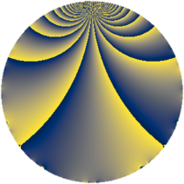# Properties

 Label 2850.2.cLevel $2850$ Weight $2$ Character orbit 2850.c Rep. character $\chi_{2850}(2849,\cdot)$ Character field $\Q$ Dimension $120$ Sturm bound $1200$

# Learn more about

## Defining parameters

 Level: $$N$$ $$=$$ $$2850 = 2 \cdot 3 \cdot 5^{2} \cdot 19$$ Weight: $$k$$ $$=$$ $$2$$ Character orbit: $$[\chi]$$ $$=$$ 2850.c (of order $$2$$ and degree $$1$$) Character conductor: $$\operatorname{cond}(\chi)$$ $$=$$ $$285$$ Character field: $$\Q$$ Sturm bound: $$1200$$

## Dimensions

The following table gives the dimensions of various subspaces of $$M_{2}(2850, [\chi])$$.

Total New Old
Modular forms 624 120 504
Cusp forms 576 120 456
Eisenstein series 48 0 48

## Trace form

 $$120q - 120q^{4} + O(q^{10})$$ $$120q - 120q^{4} + 120q^{16} - 4q^{19} - 16q^{39} - 96q^{49} + 36q^{54} + 24q^{61} - 120q^{64} + 12q^{66} + 4q^{76} + 16q^{81} + 116q^{99} + O(q^{100})$$

## Decomposition of $$S_{2}^{\mathrm{new}}(2850, [\chi])$$ into newform subspaces

The newforms in this space have not yet been added to the LMFDB.

## Decomposition of $$S_{2}^{\mathrm{old}}(2850, [\chi])$$ into lower level spaces

$$S_{2}^{\mathrm{old}}(2850, [\chi]) \cong$$ $$S_{2}^{\mathrm{new}}(285, [\chi])$$$$^{\oplus 4}$$$$\oplus$$$$S_{2}^{\mathrm{new}}(570, [\chi])$$$$^{\oplus 2}$$$$\oplus$$$$S_{2}^{\mathrm{new}}(1425, [\chi])$$$$^{\oplus 2}$$